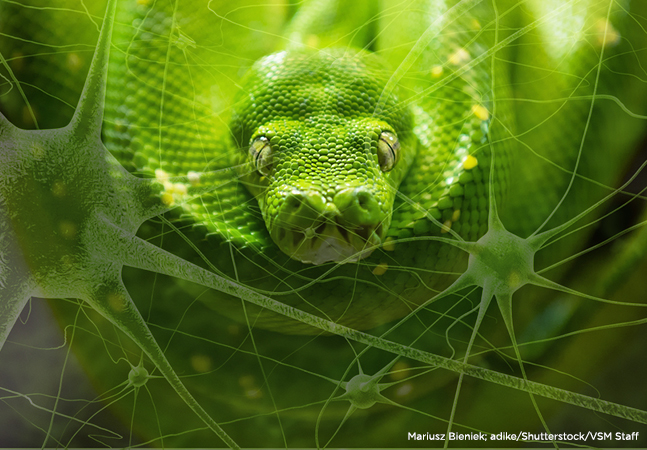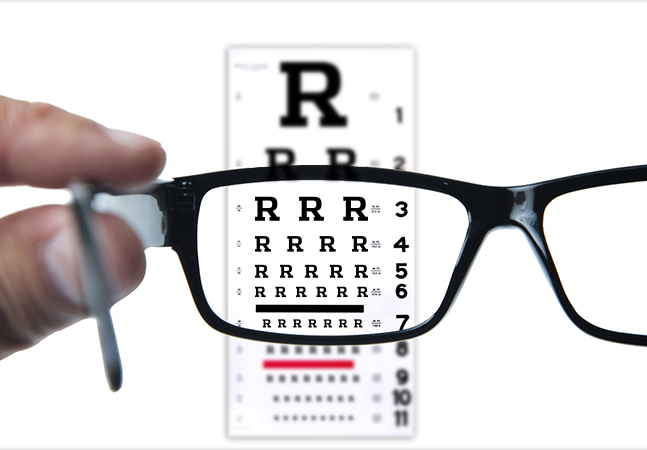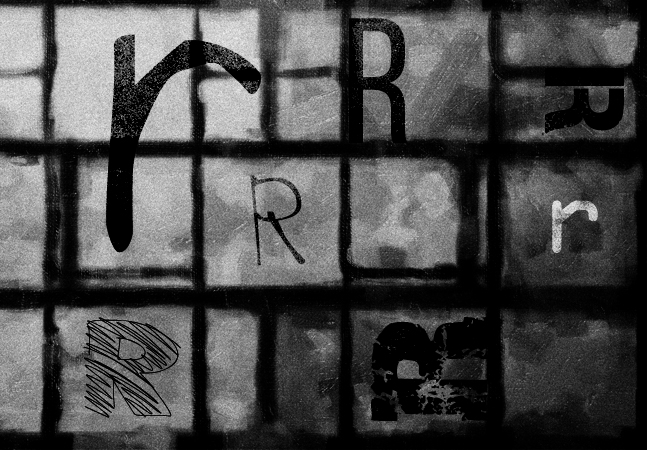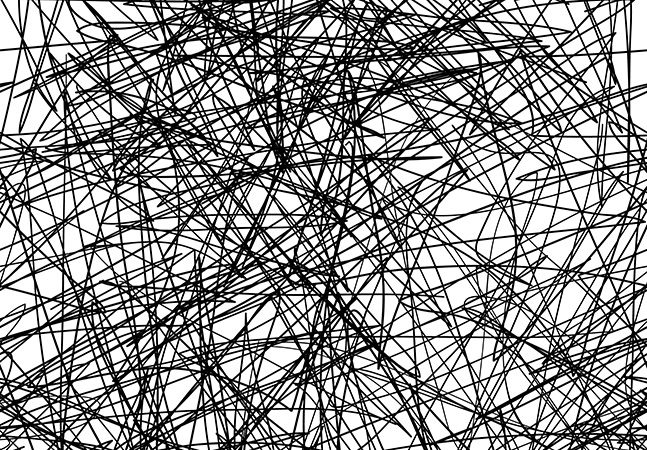The Data Science LabNeural Network Batch Training Using Python

Our resident data scientist explains how to train neural networks with two popular variations of the back-propagation technique: batch and online.Neural Network L2 Regularization Using Python

Our data science expert continues his exploration of neural network programming, explaining how regularization addresses the problem of model overfitting, caused by network overtraining.Neural Network Momentum Using Python

With the help of Python and the NumPy add-on package, I'll explain how to implement back-propagation training using momentum.Neural Network Cross Entropy Using Python

James McCaffrey uses cross entropy error via Python to train a neural network model for predicting a species of iris flower.Neural Network Back-Propagation Using Python

You don't have to resort to writing C++ to work with popular machine learning libraries such as Microsoft's CNTK and Google's TensorFlow. Instead, we'll use some Python and NumPy to tackle the task of training neural networks.Neural Networks Using Python and NumPy

With Python and NumPy getting lots of exposure lately, I'll show how to use those tools to build a simple feed-forward neural network.This R/S4 Demo Might Take You Out of Your Comfort Zone

Let's explore factor analysis again, this time using the R ability to tap into OOP, but we won't use the RC model.Revealing Secrets with R and Factor Analysis

Let's use this classical statistics technique -- and some R, of course -- to get to some of the latent variables hiding in your data.Data Clustering Using R

Find the patterns in your data sets using these Clustering.R script tricks.R Language OOP Using S3

The S3 OOP model is still widely used, so let's use write S3-style OOP code via the R language.Logistic Regression Using R

I predict you'll find this logistic regression example with R to be helpful for gleaning useful information from common binary classification problems.Neural Networks Using the R nnet Package

The R language simplifies the creation of neural network classifiers with an add-on that lays all the groundwork.Results Are in -- the Sign Test Using R

The R language can be used to perform a sign test, which is handy for comparing "before and after" data.R Language Searching and Sorting

A language that's data-intensive naturally should have a way to dig into the data effectively. Here's a look at some of the R functions for searching and sorting through it all.R Language Basic Data Structures

Vectors, lists, arrays, matrices and data frames -- a look at five of the most fundamental data structures built into R.Permutations Using R

R has limited support for mathematical permutations, but it's there. Here's what R is capable of accomplishing.How the R Language Does OOP

It's not quite like C# or Python, but the R language's object-oriented programming capabilities are getting better with each iteration. Let's take a look at what .NET developers are able do now with OOP in R6.Classic Stats, Or What ANOVA with R Is All About

New to this type of analysis? It's a classic statistics technique that is still useful. Here's a technique for doing a one-way ANOVA using R.Program-Defined Functions in R

The three most common open source technologies for writing data science programs are Python, SciLab, and R. Here's how to write program-defined functions in R.Chi-Square Tests Using R

R is the perfect language for creating a variety of chi-square tests, which are used to perform statistical analyses of counts of data. Here's how, with some sample code.Fundamentals of T-Test Using R

Linear regression was easy, right? Now, let's check out t-test analysis using R.Linear Regression with R

Now that you've got a good sense of how to "speak" R, let's use it with linear regression to make distinctive predictions.Introduction to R for .NET Developers

C# developers who want to wring more meaningful info from large sets of data should get cozy with the statistical computing language known as R. Let's get familiar with R in this new series.

Upcoming Events